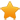Switch Editions?
CancelChannel: CodeSection,代码区,网络安全 - CodeSec
Viewing all articles

# R packaging industry close-up: How fast are we growing?

0
0

I worked a bit over the weekend preparing my talk to be delivered at the seminar organized by IBPAD this week at University of Brasilia, addressing the interfaces of Big Data and Society. I was invited to present the R package SciencesPo for an eclectic crowd. Eclectic in terms of background as well as familiarity with R, so, I thought it would be a fair introduction to call the audience’s attention to the R ecosystem, particularly, the growing number of specialized packages made available through CRAN.

I gathered some log data from package downloads to produce the following figure. The main plot shows the number of published packages since 2005 (that are still available). Notice that the y-axis is in log scale. The small multiples inside also shows the count of packages published on CRAN, but only for packages submitted after 2013-01-01. It’s an arbitrary date that makes my job of estimating a growth rate of package submission a lot easier. The red line represents the modeled growth rate estimated for the period with an approximation of 5.6%/month.

Need packages

library(ggplot2) library(grid) library(rvest) library(dplyr) library(zoo) library(SciencesPo)

Data manipulation

url <- "https://cran.r-project.org/web/packages/available_packages_by_date.html" page <- read_html(url) page %>% html_node("table") %>% html_table() %>% mutate(count = rev(1:nrow(.))) %>% mutate(Date = as.Date(Date)) %>% mutate(Month = format(Date, format = "%Y-%m")) %>% group_by(Month) %>% summarise(published = min(count)) %>% mutate(Date = as.Date(as.yearmon(Month))) -> pkgs

The main plot

gg <- ggplot(pkgs, aes(x = Date, y = published)) gg <- gg + geom_line(size = 1.5) gg <- gg + scale_y_log10( breaks = c(0, 10, 100, 1000, 10000), labels = c("1", "10", "100", "1.000", "10.000")) gg <- gg + labs(x = "", y = "# Packages (log)", title = "Packages published on CRAN ever since") gg <- gg + theme_538(base_size = 14, base_family = "Tahoma") gg <- gg + theme(panel.grid.major.x = element_blank()) gg <- gg + geom_hline(yintercept = 0, size = 1, colour = "#535353") gg ```

pkgs %>% filter(Date > as.Date("2012-12-31")) %>% mutate(publishedGrowth = c(tail(.\$published,-1), NA) / published) %>% mutate(counter = 1:nrow(.)) -> new_pkgs

Small multiples plot

gg2 <- ggplot(new_pkgs, aes(x = Date, y = published)) gg2 <- gg2 + geom_line(size = 1) gg2 <- gg2 + geom_line(data = new_pkgs, aes(y = (min(published) * 1.056 ^ counter)), color = 'red',size = .7, linetype = 1) gg2 <- gg2 + annotate("segment", x = as.Date("2014-08-01"), xend = as.Date("2014-11-01"), y = 500, yend = 500, colour = "red", size = 1) gg2 <- gg2 + annotate("text", x = as.Date("2015-10-01"), y = 500, label = "5.6% growth estimation", size = 3.5) gg2 <- gg2 + scale_y_continuous() gg2 <- gg2 + labs(y = "# Packages", x = "", title = "Packages published on CRAN since 2013") gg2 <- gg2 + theme_538(legend = "top", base_size = 11, base_family = "Tahoma", colors = c("gray97", "#D2D2D2", "#2b2b2b", "#2b2b2b")) gg2 <- gg2 + theme(panel.grid.major.x = element_blank()) gg2 <- gg2 + geom_hline(yintercept = 0, size = .6, colour = "#535353") gg2 gg print(gg2, vp=viewport(.775, .31, .43, .43))

← Previous Post

Viewing all articles

More Pages to Explore .....